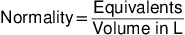##### Chemistry Workbook For Dummies with Online Practice, 3rd EditionIn the world of chemistry, not all acids and bases are created equally. Some have an innate ability to neutralize more effectively than others.

Consider hydrochloric acid (HCl) and sulfuric acid (H2SO4), for example. If you mixed 1 M sodium hydroxide (NaOH) together with 1 M hydrochloric acid, you'd need to add equal amounts of each to create a neutral solution of sodium chloride (NaCl) and water (H2O). If you mixed sodium hydroxide with sulfuric acid, however, you'd need to add twice as much sodium hydroxide as sulfuric acid to create a solution of sodium sulfate (Na2SO4) and water.

Why this blatant inequality of acids? The answer lies in the balanced neutralization reactions for both acid/base pairs:

HCl + NaOH → NaCl + H2O

H2SO4 + 2NaOH → Na2SO4 + 2H2O

The coefficients in the balanced equations are the key to understanding this inequality. To balance the second equation, the coefficient 2 needs to be added to sodium hydroxide, indicating that 2 mol of it must be present to neutralize 1 mol of sulfuric acid.

On a molecular level, this happens because sulfuric acid has two acidic hydrogen atoms to give up, and the single hydroxide in a molecule of sodium hydroxide can neutralize only one of those two acidic hydrogens to form water. Therefore, 2 mol of sodium hydroxide are needed for every 1 mol of sulfuric acid.

Hydrochloric acid, on the other hand, has only one acidic hydrogen to contribute, so it can be neutralized by an equal amount of sodium hydroxide, which has only one hydroxide to contribute to neutralization.

The number of moles of an acid or base multiplied by the number of hydrogens or hydroxides that a molecule has to contribute in a neutralization reaction is called the number of equivalents of that substance.

Basically, the number of effective neutralizing moles available determines the ratio of acid to base in a neutralization reaction. In the hydrochloric acid example, there's 1 equivalent of acid (from HCl) present and 1 equivalent of hydroxide (from NaOH) present.

In the second example, there are 2 equivalents of acid (from H2SO4) and 2 equivalents of hydroxide present (from NaOH and the coefficient of 2). In chemistry life, this idea can come in quite handy when you want to neutralize an acid or base for disposal or cleanup.

In addition to dealing with the more common concept of molarity, you may encounter a concentration measure called normality, which is simply the number of equivalents divided by the volume in liters:As you can see from the formula, molarity and normality are very similar. Normality, however, takes equivalents into account. Mixing equal amounts of acidic and basic solutions of equal normality always results in a neutral solution, while the same can't be said of solutions of equal molarity.